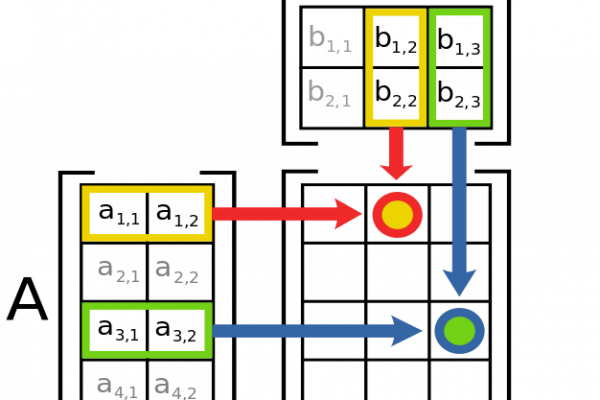# Introduction to Matrices and Matrix Arithmetic for Machine LearningLast Updated on August 9, 2019

Matrices are a foundational element of linear algebra.

Matrices are used throughout the field of machine learning in the description of algorithms and processes such as the input data variable (X) when training an algorithm.

In this tutorial, you will discover matrices in linear algebra and how to manipulate them in Python.

After completing this tutorial, you will know:

• What a matrix is and how to define one in Python with NumPy.
• How to perform element-wise operations such as addition, subtraction, and the Hadamard product.
• How to multiply matrices together and the intuition behind the operation.

Kick-start your project with my new book Linear Algebra for Machine Learning, including step-by-step tutorials and the Python source code files for all examples.

Let’s get started.

• Update Jun/2019: Fixed a typo in the Matrix-Vector Multiplication section (thanks M. Vincent).
• Update Jun/2019: Fixed typo in description of matrix in Python (thanks Ari).A Gentle Introduction to Matrices for Machine Learning
Photo by Maximiliano Kolus, some rights